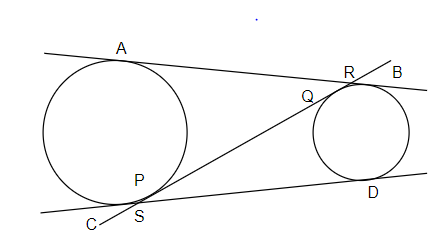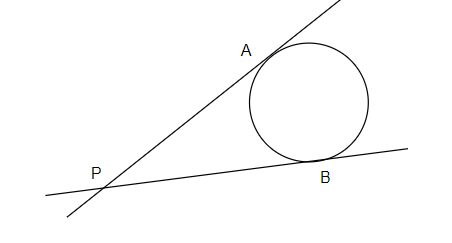QUESTION

# Suppose ${{S}_{1}}$and ${{S}_{2}}$are two unequal circles; AB and CD are the direct common tangents to the circles. A transverse common tangent PQ cuts AB in R and CD in S. If AB=10, then RS is?a)8b)9c)10d)11Hint: We will use the property that the tangent from some point to the circle has some length. The length of PA is equal to the length of PB because the two tangents from a point to the circle are equal.it is given in the question that S1 and S2 are two unequal circles. Also, AB and CD are the direct common tangents to the circles. It is also given that a transverse common tangent PQ cuts AB in R and CD in S, and length of AB=10 then we have to find the length of RS.We know that the length of two tangents from a point to the circle are of equal length. So, we get $RQ=RB$ and $SC=SP$. Let us assume that $RQ=RB=x$ and $SC=SP=y$. Now, we know that the length of AB is 10 units, so we get length $AR=AB-RB=RP=10-x$. AR and RP are the two tangents from a common point R to the circle S1 thus are of equal length.

As we have $AR=AB-RB=RP=10-x$ and $RQ=x$, so from this we get $PQ=RP-RQ=10-x-x$ or $PQ=10-2x$. Again the tangents SQ and SD are equal because both are common on point S and circle ${{S}_{2}}$, and also we had assumed that $SP=y$ and we have already calculated $PQ=10-2x$. Therefore, we get $SQ=SP+PQ=y+10-2x=SD$.

We also know that the length of direct common tangents of two circles are equal thus tangents $AB=CD=10$. Now, we get $CD=CS+SD$ and we have $CD=10,CS=y$ and $SD=10-2x+y$. On putting the value of CD, CS and SD, we get $10=y+10-2x+y$. On solving, we get $2y=2x$ or $x=y$.

Therefore, $RS=RO+PQ+PS$, we have $RQ=x$, $PQ=10-2x$ and $PS=y$, thus we get $RS=x+10-2x+y$, solving further by taking $x=y$, we get $RS=10$. Thus, the length of RS is 10 and option c) is correct.

Note: The question becomes very easy if we know the property that the length of two tangents from a point to a circle are equal. It can be proved easily using the concept of congruence of triangles.